# Class 12 Maths NCERT Solutions for Chapter 7 Integrals Exercise 7.5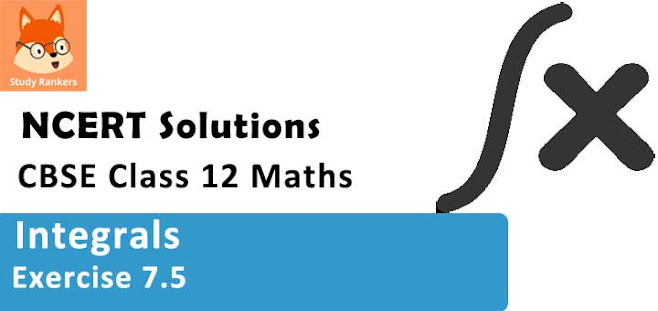### Integrals Exercise 7.5 Solutions

1. Integrate the rational functions x/[(x + 1)(x + 2)]

Solution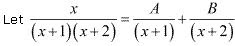⇒ x = A(x + 2) + B(x + 1)
Equating the coefficients of x and constant term, we obtain
A + B = 1
2A + B = 0
On solving, we obtain
A = -1 and B = 2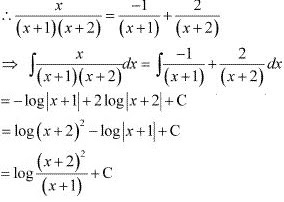2. Integrate the rational functions 1/(x2 – 9)
Solution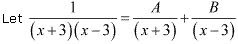I = A(x - 3) + B(x + 3)
Equating the coefficients of x and constant term, we obtain
A + B = 0
−3A + 3B = 1
On solving, we obtain
A = -1/6 and B = 1/6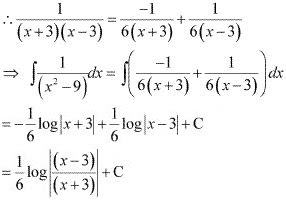3. Integrate the rational functions (3x - 1)/[(x - 1)(x - 2)(x - 3)]
Solution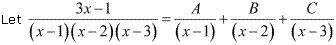3x - 1 = A(x - 2)(x - 3) + B(x - 1)(x - 3) + C(x - 1)(x - 2)  ...(1)
Substituting x = 1, 2, and 3 respectively in equation (1), we obtain
A = 1, B = -5, and C = 4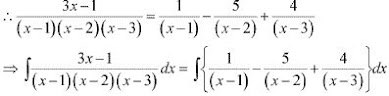= log|x - 1| - 5 log|x - 2| + 4 log|x - 3| + C

4. Integrate the rational functions x/[(x - 1)(x - 2)(x -3)]
Solution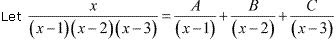x = A(x - 2)(x - 3) + B(x - 1)(x - 3) + C(x - 1)(x - 2)  ...(1)
Substituting x = 1, 2, and 3 respectively in equation (1), we obtain
A = 1/2, B = -2, and C = 3/2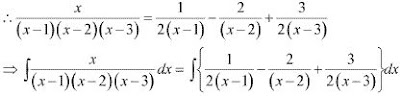= (1/2) log|x - 1| - 2 log|x - 2| + (3/2) log |x - 3| + C

5. Integrate the rational functions 2x/(x2 + 3x + 2)
Solution
Let 2x/(x2 + 3x + 2) = A/(x + 1) + B/(x + 2)
2x = A(x + 2) + B(x + 1)  ...(1)
Substituting x = -1 and -2 in equation (1), we obtain
A = -2 and B = 4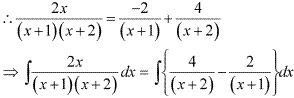= 4 log|x + 2| - 2log|x + 1| + C

6. Integrate the rational functions (1 - x2 )/x(1 - 2x)
Solution
It can be seen that the given integrand is not a proper fraction.
Therefore, on dividing (1 - x2 ) b x(1 - 2x), we obtain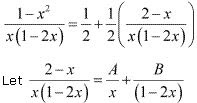⇒ (2 - x) = A(1 - 2x) + Bx  ...(1)
Substituting x = 0 and 1/2 in equation (1), we obtain
A = 2 and B = 3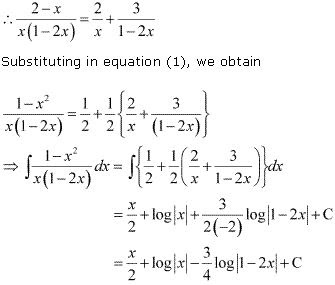7. Integrate the rational functions x/[(x2 + 1)(x - 1)]
Solution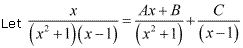x = (Ax + B)(x - 1) + C(x2 + 1)
x = Ax2 - Ax + Bx - B + Cx2 + C
Equating the coefficients of x2 , x, and constant term, we obtain
A + C = 0
-A + B = 1
-B + C = 0
On solving these equations, we obtain
A = -1/2, B = 1/2, and C = 1/2
From equation (1), we obtain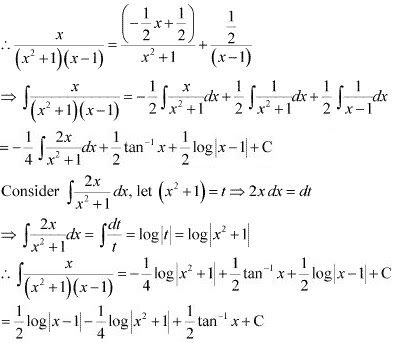8. Integrate the rational functions x/[(x - 1)2 (x + 2)]
Solution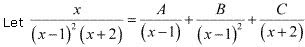x = A(x - 1)(x + 2) + B(x + 2) + C(x - 1)2
Substituting x = 1, we obtain
B = 1/3
Equating the coefficients of x2 and constant term, we obtain
A + C = 0
-2A + 2B + C = 0
On solving, we obtain
A = 2/9 and C = -2/9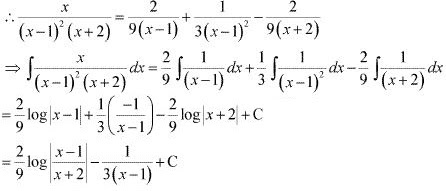9. Integrate the rational functions (3x + 5)/(x3 - x2 - x + 1)
Solution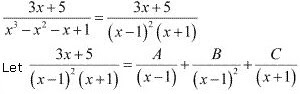3x + 5 = A(x- 1)(x + 1) + B(x + 1) + C(x - 1)2
3x + 5 = A(x2 - 1) + B(x + 1) + C(x2 + 1 - 2x)  ...(1)
Substituting x = 1 in equation (1), we obtain
B = 4
Equating the coefficients of x2 and x, we obtain
A + C = 0
B - 2C = 3
On solving, we obtain
A = -1/2 and C = 1/2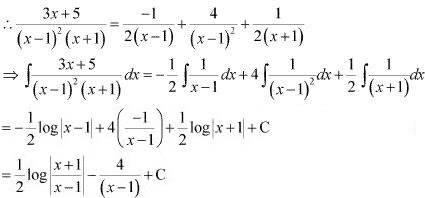10. Integrate the rational functions (2x - 3)/[(x2 - 1)(2x  + 3)]
Solution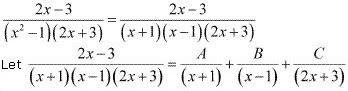⇒ (2x - 3) = A(x - 1)(2x + 3) + B(x + 1)(2x + 3) + C(x + 1)(x - 1)
⇒ (2x - 3) = A(2x2 + x - 3) + B(2x2 + 5x + 3) + C(x2 - 1)
⇒ (2x - 3) = (2a + 2B + C)x2 + (A + 5B)x + (-3A + 3B - C)
Equating the coefficients of x2 and x, we obtain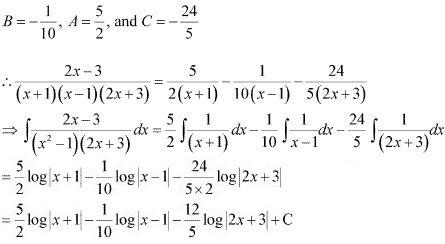11. Integrate the rational functions 5x/[(x + 1)(x2 - 4)]
Solution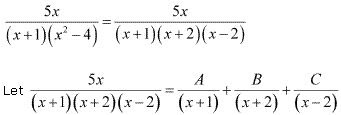5x = A(x + 2)(x - 2) + B(x + 1)(x - 2) + C(x + 1)(x + 2) ...(1)
Substituting x = -1, -2 and 2 respectively in equation (1), we obtain
A = 5/3, B = -5/2, and C = 5/6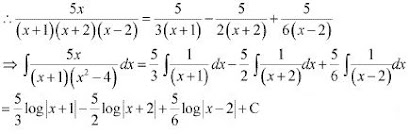12. Integrate the rational functions (x2 + x + 1)/(x2 - 1)
Solution
It can be seen that the given integrand is not a proper fraction.
Therefore, on dividing (x3 + x + 1) by x2 - 1, we obtain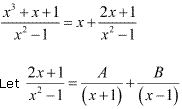2x + 1 = A(x - 1) + B(x + 1) ...(1)
Substituting x = 1 and -1  in equation (1), we obtain
A = 1/2 and B = 3/2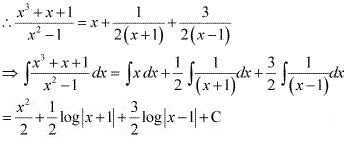13. Integrate the rational functions 2/[(1 - x)(1 + x2 )]
Solution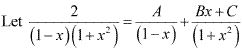2 = A(1 + x2 )+ (Bx + C)(1 - x)
2 = A + Ax2 + Bx - Bx2 + C - Cx
Equating the coefficient of x2 , x, and constant term, we obtain
A - B = 0
B - C = 0
A + C = 2
On solving these equations, we obtain
A = 1, B = 1, and C = 1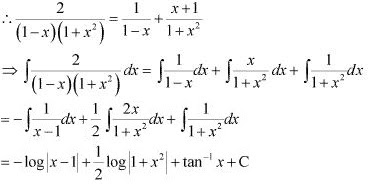14. Integrate the rational functions (3x - 1)/(x + 2)2
Solution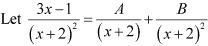⇒ 3x - 1= A(x + 2) + B
Equating the coefficient of x and constant term, we obtain
A = 3
2A + B = -1
⇒ B = -7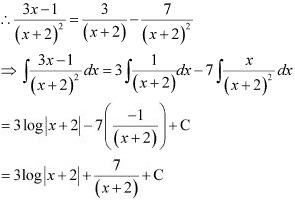15. Integrate the rational functions 1/(x4 - 1)
Solution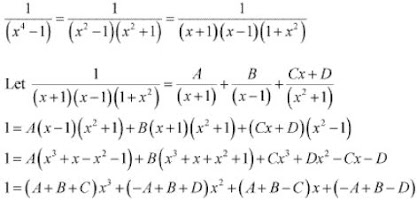Equating the coefficient of x3, x2, x and constant term, we obtain
A + B + C = 0
-A + B + D = 0
A + B - C = 0
-A + B - D = 1
On solving these equations, we obtain
A= -1/4, B =1/4, C = 0 and D = -1/2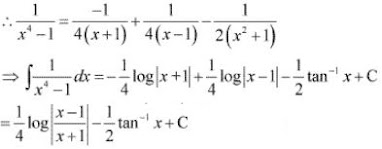16. Integrate the rational functions 1/[x(xn + 1)] [Hint : multiply numerator and denominator by xn - 1 and put xn = t] .
Solution
1/[x(xn + 1)]
Multiplying numerator and denominator by xn - 1  , we obtain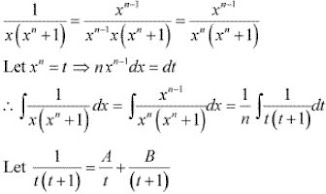1 = A(1 + t) + Bt  ...(1)
Substituting t = 0, -1 in equation (1), we obtain
A = 1 and B = -1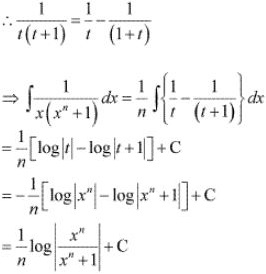17. Integrate the rational functions cos x/[(1 - sin x)(2 - sin x)  [Hint : Put sin x = t]
Solution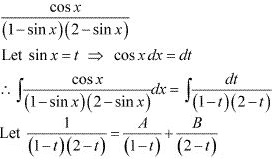l = A(2 - t) + B(1 - t)
Substituting t = 2 and then t = 1 in equation (1), we obtain
A = 1 and B = -1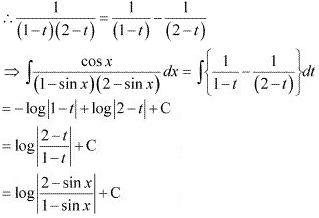18. Integrate the rational functions [(x2 + 1)(x2 + 2)]/[(x2 + 3)(x2 + 4)]
Solution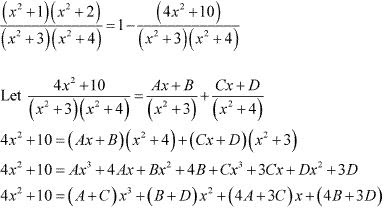Equating the coefficients of x3 , x2 , x, and constant term, we obtain
A + C = 0
B + D = 4
4A + 3C = 0
4B + 3D = 10
On solving these equations, we obtain
A = 0, B = -2, C = 0, and D = 6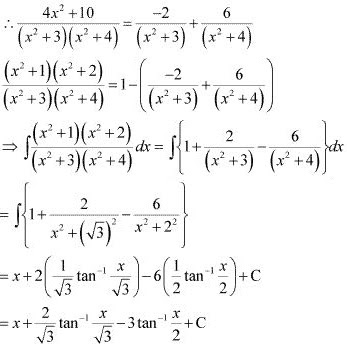19. Integrate the rational functions 2x/[(x2 + 1)(x2 + 3)]
Solution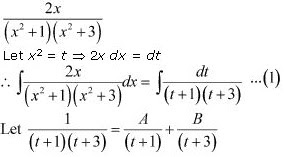l = A(t + 3) + B(t + 1) ...(1)
Substituting t = -3 and t = -1 in equation (1), we obtain
A = 1/2 and B = -1/2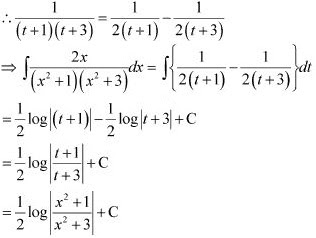20. Integrate the rational functions 1/[x(x4 - 1)]
Solution
1/[x(x4 - 1)]
Multiplying numerator and denominator by x3 , we obtain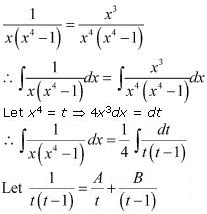l = A (t - 1) + Bt  ...(1)
Substituting t = 0 and 1 in (1), we obtain
A = -1 and B = 1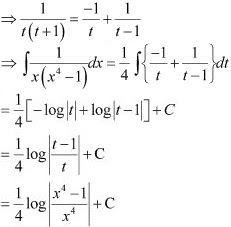21. Integrate the rational functions 1/(ex - 1)  [Hint : Put ex = t] .
Solution
1/(ex - 1)
Let ex = t ⇒ ex dx = dt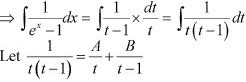l = A (t - 1) + Bt  ...(1)
Substituting t = 1 and t = 0 in equation (1), we obtain
A = -1 and B = 1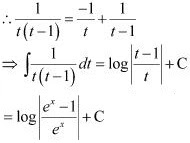22. Choose the correct answer ∫ xdx/[(x - 1)(x - 2)]  equals
A. log |(x - 1)2 /(x - 2)| + C
B. log |(x - 2)2 /(x - 1)| + C
C. log|[(x - 1)/(x - 2)]2 | + C
D. log |(x - 1)(x - 2)| + C
Solution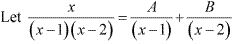x = A(x - 2) + B(x - 1) ...(1)
Substituting x = 1 and 2 in (1), we obtain
A = -1 and B = 2
Hence, the correct answer is B.

23. Choose the correct answer ∫ dx/[x(x2 + 1)] equal
A. log|x| - (1/2) log (x2 + 1) + C
B. log|x| + (1/2)log (x2 + 1) + C
C. - log |x| + (1/2) log(x2 + 1) + C
D. (1/2) log |x| + log (x2 + 1) + C
Solution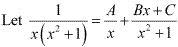l = A(x2 + 1) + (Bx + C)x
Equating the coefficients of x2, x and constant term, we obtain
A + B = 0
C =  0
A = 1
On solving these equations, we obtain
A = 1, B = -1, and C = 0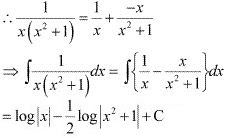Hence, the correct answer is A.